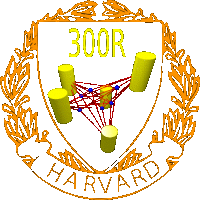Omni 3D project
300R: Mathematics of 3D image synthesis
Software
Office: SciCtr 434
 demo_statistics.m is a Mathematica program which allows to investigate the error of reconstruction for syntetic random data with arbitrary many spherical cameras in space and arbitrarily many points. An error is added to the syntetic pictures and then the reconstruction done. It is one of the programs we used to experimentally investigate the stability of the reconstruction. simulation3d_vel.m is a Mathematica program which demonstrates the reconstruction of camera and point particles as particle velocities from pictures. Having the possiblity to reconstruct moving bodies as well as the cameras is a justification for a relatively general definition of the structure from motion problem. Ullman's Theorem in two dimensions (Wolfram Demonstrations project) ullman3d.nb Ullmans Theorem in three dimensions, Mathematica demonstration, not submitted yet to the Mathematica demonstration project